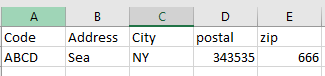# Retriving data from an excel on basis of multiple columns

I have an excel sheeton the basis of Column B,C,D and E i need to retrive Value from column A that is ABCD

Hi

You can use linq query here

``````resultArray = dt.AsEnumerable().
Where(Function(row) row.Field(Of String)("B") = "ConditionB" AndAlso
row.Field(Of String)("C") = "ConditionC" AndAlso
row.Field(Of String)("D") = "ConditionD" AndAlso
row.Field(Of String)("E") = "ConditionE").
Select(Function(row) row.Field(Of String)("A")).ToArray()

resultString = String.Join(", ", resultArray)
``````

Hope this helps

Cheers @Pogboom

Based on the what condition we need to get the value from that because you mentioned B,C,D And E so

@Pogboom

what will be the variable type for this resultArray one any specific?

Resultarray will be of type array of string
@Pogboom

resultString = String.Join(", ", resultArray)
what does this conidtion do?

This concatenates all the values in an array to a string
Comma is the delimiter between each value in a string

Say array has {1,2,3}
Then this join would do
“1, 2, 3”

@Pogboom

just need to fetch value from first column on basis on or more like checks on B,C,D and E

any other approach i am getting error : unable to cast object of type system.double to system.string

this is not working getting error like unable to cast objectof type system.double to system.string

1 Like

I think it’s because some of the column has numerical value
Simply mention like this in linq query

``````resultArray = dt.AsEnumerable().
Where(Function(row) row("B").ToString.Equals ("ConditionB") AndAlso row("C").ToString.Equals("ConditionC") AndAlso row("D").ToString.Equals("ConditionD") AndAlso row("E").ToString.Equals("ConditionE")
Select(Function(row) row("A")).ToArray()

resultString = String.Join(", ", resultArray)
``````

Just removed to string field type
Cheers @Pogboom

@Pogboom

`dt.AsEnumerable.Where(function(x) x("Address").ToString.Equals(AddressVariable) and x("City").ToString.Equals(CityVariable) and x("Postal").ToString.Equals(PostalVariable.ToString) and x("Zip").ToString.Equals(ZipVariable)).Select(function(x) x("Code").ToString).FirstOrDefault`

cheers

what will be the variable type?

@Pogboom

it is string

cheers

if there are no headers only value in an excel what will change in query?

@Pogboom

use indexes liek 0,1,2,3 instead of columnName

cheers